# Adiabatic process facts for kids

Kids Encyclopedia Facts

An adiabatic process is a thermodynamic process where a fluid becomes warmer or cooler without getting heat from, or giving it to, something else. Usually the temperature instead changes because of changes in pressure.

Adiabatic cooling is the usual cause of clouds. When warm, humid air rises due to convection or other cause, water condenses to make clouds, and in some cases precipitation. Convection also causes cold air to sink. This warms it adiabatically, often destroying a cloud and sometimes causing any precipitation to evaporate before hitting the ground.

## Description

If we take some fluids in an insulated system and expand or compress the system very fast, the system won't be able to gain or loose any heat (from outside of the system). (In reality, you can't get a perfectly insulated system. But in some cases, the heat transfer is so low that we can call them adiabatic process.)

From the first law of thermodynamics, we know that,,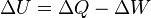$\Delta U = \Delta Q - \Delta W$

Here,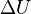$\Delta U$ is the change of internal energy;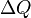$\Delta Q$ is the change of heat and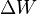$\Delta W$ is the change of work.

Since there occurs no heat transfer in adiabatic process, the$\Delta Q$ becomes 0. So, for adiabatic process, the equation is,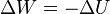$\Delta W = -\Delta U$

Again, we know that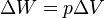$\Delta W = p\Delta V$.(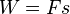$W = F s$;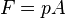$F = p A$;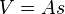$V = A s$; so,$\Delta W = p\Delta V$)

Here,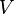$V$ is volume;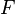$F$ is force;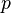$p$ is pressure;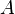$A$ is the area and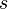$s$ is the distance.

So, the equation stands as,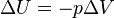$\Delta U = -p\Delta V$

Which means, by doing work (changing volume), one can change the internal energy (and so the temperature) in a adiabatic process.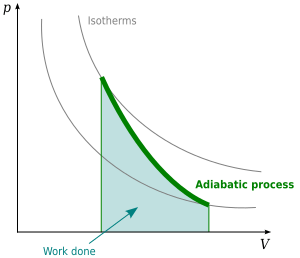In adiabatic process, increasing the volume will result in decrease of the internal energy

In the case of Adiabatic process (see the upper section),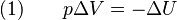$\text{(1)} \qquad p\Delta V = -\Delta U$

Again, in constant volume, the heat capacity of one mole gas is,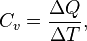$C_v = \frac{\Delta Q}{\Delta T},$

Here,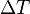$\Delta T$ is the change of temperature and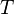$T$ is the temperature।

But, in constant volume (as no work is done),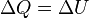$\Delta Q = \Delta U$

So,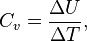$C_v = \frac{\Delta U}{\Delta T},$

From the first (1) equation,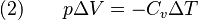$\text{(2)} \qquad p\Delta V = -C_v\Delta T$

Now, the Ideal gas law for one mole gas is,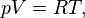$pV = RT,$

Here,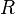$R$ is the gas constant.

By differentiating the last equation,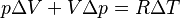$p\Delta V + V\Delta p = R\Delta T$

Or,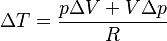$\Delta T = \frac{p\Delta V + V\Delta p}{R}$

Now from equation (2), we get,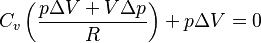$C_v \left( \frac{p\Delta V + V\Delta p}{R} \right) + p\Delta V= 0$

Since,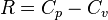$R = C_p - C_v$ (Mayer's relation),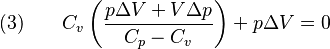$\text{(3)} \qquad C_v \left( \frac{p\Delta V + V\Delta p}{C_p - C_v} \right) + p\Delta V= 0$

Here,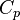$C_p$ is the heat capacity of gas at constant pressure.

Simplifying the equation (3),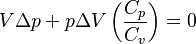$V\Delta p + p\Delta V \left(\frac{C_p}{C_v}\right) = 0$

If,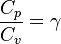$\frac{C_p}{C_v} = \gamma$,

Then,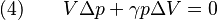$\text{(4)} \qquad V\Delta p + \gamma p\Delta V = 0$

By dividing both sides of the equation (4) with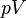$pV$ we get,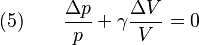$\text{(5)} \qquad \frac{\Delta p}{p} + \gamma \frac{\Delta V}{V} = 0$

Integrating (Integration leaves a constant) the equation (5),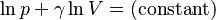$\ln p + \gamma \ln V = \text{(constant)}$

If we assume that,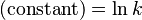$\text{(constant)} = \ln k$,

We get,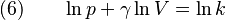$\text{(6)} \qquad \ln p + \gamma \ln V = \ln k$

Simplifying the equation (6),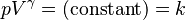$p V^\gamma = \text{(constant)} = k$

This is the relation between pressure and volume in adiabatic process.In Spanish: Proceso adiabático para niños